•••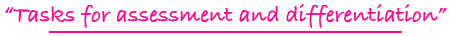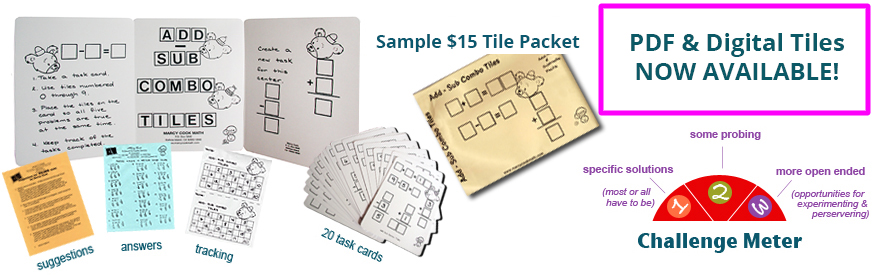Tiling Task Card Packets include 20 unique task cards on card stock,  3 backdrop cards , tracking sheets, suggestions and answer sheets.  These are contained in a printed manila envelope for convenient storing.  A set of number tiles 0 - 9 is  needed for each task card

2 - 4

### MULTI-REP DIGITAL TILES

forming multiplication equations from representational models: rectangular arrays, intersecting lines, dot arrays, and pictures

Challenge Level: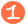DIGITAL FORMAT\$10.00

2 - 4

### MULTI-REP TILES

forming multiplication equations from representational models: rectangular arrays, intersecting lines, dot arrays, and pictures

Challenge Level:PDF FORMAT
(You will receive both color and black and white files for printing.)\$10.00

4 - 8

### MULTIPLE/FACTOR DIGITAL TILES

finding multiples, including least common multiple, and factors, including greatest common factor

Challenge Level: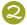DIGITAL FORMAT\$10.00

4 - 8

### MULTIPLE/FACTOR TILES

finding multiples, including least common multiple, and factors, including greatest common factor

Challenge Level:PDF FORMAT
(You will receive both color and black and white files for printing.)\$10.00

3 - 6

### MULTIPLICATION FACT DIGITAL TILES

using a rectangular array as a representational model to form multiplication fact equations

Challenge Level:DIGITAL FORMAT\$10.00

3 - 6

### MULTIPLICATION FACT TILES

using a rectangular array as a representational model to form multiplication fact equations

Challenge Level:PDF FORMAT
(You will receive both color and black and white files for printing.)\$10.00

4 - 7

### MULTIPLICATION/DIVISION TILES

completing multiplication and division equations and dealing with factors of numbers and multiples of numbers

Challenge Level:\$15.00

4 - 7

### MULTIPLICATION/DIVISION DIGITAL TILES

completing multiplication and division equations and dealing with factors of numbers and multiples of numbers

Challenge Level:DIGITAL FORMAT\$10.00

4 - 7

### MULTIPLICATION/DIVISION TILES

completing multiplication and division equations and dealing with factors of numbers and multiples of numbers

Challenge Level:PDF FORMAT
(You will receive both color and black and white files for printing.)\$10.00

4 - 6

### MULTIPLY TO A PRODUCT DIGITAL TILES

forming basic multiplication facts with 2 or 3 factors to a given product

Challenge Level:DIGITAL FORMAT\$10.00

4 - 6

### MULTIPLY TO A PRODUCT TILES

forming basic multiplication facts with 2 or 3 factors to a given product

Challenge Level:PDF FORMAT
(You will receive both color and black and white files for printing.)\$10.00

5 - 8

### MULTIPLY: THINK & TILE

forming a multiplication problem with a 4-digit number as a factor and a single-digit number as a factor by utilizing hints dealing with math concepts and vocabulary

Challenge Level: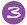\$15.00

5 - 8

### MULTIPLY: TRIAL & TILE

forming a multiplication problem where a 3-digit number is multiplied by a 2-digit number

Challenge Level:\$15.00

5 - 8

### MULTIPLY: TRIAL & TILE DIGITAL TILES

forming a multiplication problem where a 3-digit number is multiplied by a 2-digit number

Challenge Level:DIGITAL FORMAT\$10.00

5 - 8

### MULTIPLY: TRIAL & TILE

forming a multiplication problem where a 3-digit number is multiplied by a 2-digit number

Challenge Level:PDF FORMAT
(You will receive both color and black and white files for printing.)\$10.00

4 - 8

### NEGATIVE/POSITIVE INTEGER TILES

adding and subtracting of positive and negative whole numbers

Challenge Level:\$15.00

2 - 5

### NUMBER SENSE TILES

forming specified numbers using math vocabulary and concepts

Challenge Level:\$15.00

3 - 6

### NUMBER SEQUENCE DIGITAL TILES

sequencing numbers with patterns of plus, minus, or multiples in ascending and descending order

Challenge Level:DIGITAL FORMAT\$10.00

3 - 6

### NUMBER SEQUENCE TILES

sequencing numbers with patterns of plus, minus, or multiples in ascending and descending order

Challenge Level:PDF FORMAT
(You will receive both color and black and white files for printing.)\$10.00

4 - 8

### NUMBER SQUARED TILES

squaring the first ten counting numbers; pictorial and abstract

Challenge Level:\$15.00